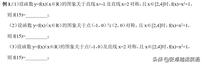# 综合使用几种常见的奇偶性、对称性和周期性函数(1) 若f(x)关于x=a对称，且关于x=b也对称(a≠b)，则f(x) 是一个循环函数，周期为2|a-b|;

(2) 若f(x)关于点(a,0)对称，也关于点(b,0)对称(a≠b)，则f(x)为周期函数，周期为2 |a-b |;

(3) 若f(x)关于点(a,0)和关于直线x=b(a≠b)对称，则f(x)是a 周期为 4|a-b| 的周期函数。

(1) 若f(x)关于直线x=a对称，则f(x )=f(2a-x) 或 f(x a)=f(a-x) 成立；

(2) 若f(x)关于点(a,0)对称，则f(x )=-f(2a-x) 或 f(x a)=-f(a-x) 成立。

(2) 解法一：f(x的像)关于点(-1, 0)和(2, 0)对称，所以f(x)是周期为6的周期函数， 则f(15)=f(15-6×2)= f(3)=10。

(3) 解一：f(x)的图像关于点(-1, 0)和直线x=2对称，所以f(x) 是周期函数 周期为12，则f(15)=f(3)=10。

(2) f(x)的图像关于原点和(2, 0)对称，所以f(x)是奇函数，f(x)是周期函数，a 2×2=4的周期，则f(17)=f(17-4×3)=f(5)=26。

(3) f(x)的图像是关于 原点与直线x=1对称，可得f(x)为奇函数，f(x)为周期函数，周期为4×1=4，则f(15)=f (15-4×3)=f(3)=- f(-3)=-f(5)=-26.

(4) 函数y=f(x)的图像 (x∈R)关于直线x=0与点(1, 0)对称，f(x)为偶函数，f(x)为周期函数，周期为4，则f( 15)=f(15-4×3)=f(3)=f(- 3)=f(5)=26。

3. 平移对称性

(1) 若f(x a)为偶函数，则f(x)的像关于直线x=a对称；

(2 ) 如果 f(x a) 是奇函数，则 f(x) 的像关于点 (a, 0) 对称

A. f(x) 是偶函数 B。f(x) 是奇函数 C。f(x)＝f (x＋2) D. f( x＋3)是一个奇函数